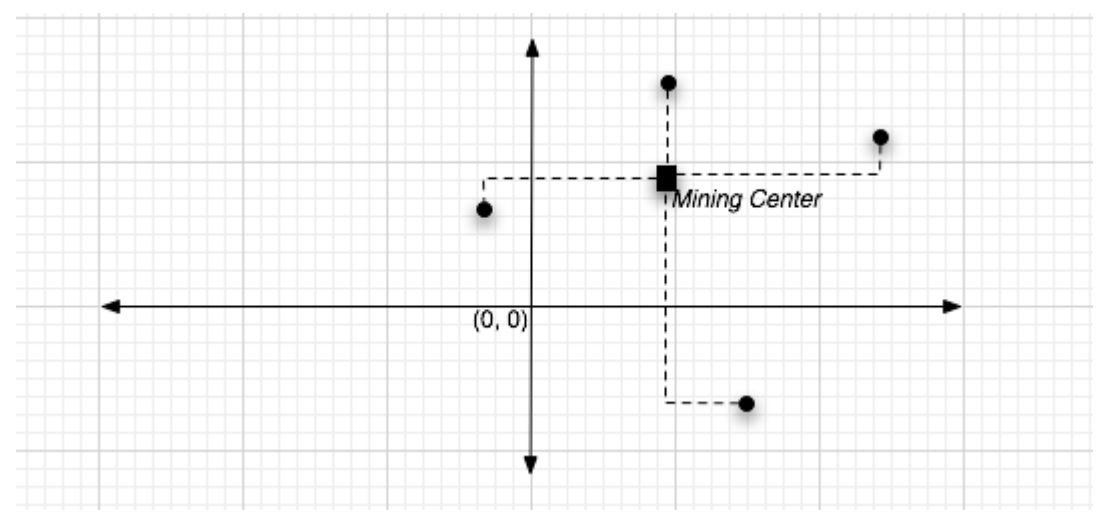시간 제한 메모리 제한 제출 정답 맞은 사람 정답 비율
1 초 128 MB 0 0 0 0.000%

## 문제

As part of a peace treaty with the Navi, the humans are allowed mine for Unobtainium in a remote, deserted area of Pandora. The scientists have identified many possible excavation sites, and are now trying to figure out where to place the Mining Center. The placement of the mining center is further constrained by the fact that the robots that will carry the Unobtainium from the excavation sites to the mining center can only walk along prespecified Grid lines (see figure). The goal is now to find the location of the mining center so that the maximum distance to the excavation sites is minimized. You are to write a program for finding that location.

Specifically, you are given the x- and y-coordinates of the excavations sites, $(x_1, y_1),(x_2, y_2), \dots,(x_n, y_n)$ ($n$ denotes the number of excavation sites). You are to find the coordinates for the Mining Center, $(x_0, y_0)$, so that the maximum of the distances between the mining center and the excavation sites is minimized. All coordinates must be integers. The distance metric to be used is the rectilinear distance (also called Manhattan distance). Specifically, the rectilinear distance between $(x_0, y_0)$ and $(x_i, y_i)$ is: $$|x_i − x_0| + |y_i − y_0|$$

Further, if there are multiple locations which qualify, then you must find the location that is closest to the origin by Euclidean distance metric. Since origin is at (0, 0), this corresponds to minimizing $\sqrt{x_0^2+y_0^2}$.In other words, given the input $(x_1, y_1), \dots,(x_n, y_n)$, your goal is to find $(x_0, y_0)$ such that: $$\max_{i}{(|x_i-x_0| + |y_i-y_0|)}$$ is minimized, and if there are multiple answers, then $\sqrt{x_0^2+y_0^2}$ is the smallest among all such answers.

## 입력

The first line in the test data file contains the number of test cases, n. After that, each line contains one test case. The test case begins with the number of mines, followed by the x and y coordinates of each mine. All coordinates must be integers. Very large coordinate values may be used (million+), so brute force methods will not work.

## 출력

For each test case, you are to output a line beginning with ”LOCATION”, followed by the x and y coordinates of the mining center. All coordinates must be integers.

## 예제 입력 1

2
4 100 0 40 0 -10 0 20 0
3 245 692 -772 -647 330 526


## 예제 출력 1

LOCATION 45 0
LOCATION -121 -120